# Activity: A Walk in the Desert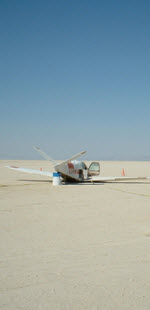## Crash!

Jade has crash-landed in the desert.

There is a village somewhere nearby, direction unknown.

So Jade comes up with a cunning plan:

• Fill up a water bottle from the plane, and take a compass,
• Then walk 1 km north, change direction and walk 2 km east, then 3 km south, 4 km west, 5 km north, 6 km east, and so on, like this: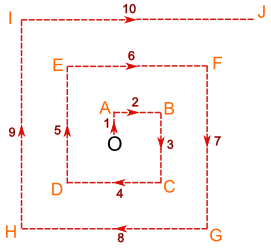This way he will find the village no matter what direction it is in, and can (hopefully) find his way back to the plane for fresh water and shade when he needs it.

But he needs to know, at the end of each stage:

1. How far he has walked altogether
2. How far (in a straight line) back to the plane

## OK, Let's Do the Calcs ...

After one stage of the journey, Jade has reached point A:

• Jade has walked 1 km altogether.
• He is 1 km (in a straight line) from the plane.

After two stages, he has reached point B:

• Jade has walked 3 km altogether.
• To answer the second question, we make a right-angled triangle OAB: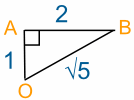We can calculate the length of OB using Pythagoras' Theorem, as follows:

OB2 = OA2 + AB2
OB2 = 12 + 22
OB2 = 1 + 4
OB2 = 5
OB = √5

So the answer in this case is:

At Point B, the distance back to the plane (in a straight line) is √5 km

After three stages, he has reached point C:

Fill in all the other values ... if it gets hard, read below for some help

Point Distance
walked altogether
Distance (in a
straight line) from O
O 0 0
A 1 1
B 3 √5
C 6
D
E
F
G
H
I
J

## How To Make It Easier

### Distance walked altogether

At the end of each stage, the total distance is the sum of the series 1 + 2 + 3 + 4 + 5 + 6 + ...

So just add the new distance each time.

OR you can calculate each value using:

n(n + 1)/2
where n is the number of stages.

Like this:

 Number of stages (n) Total distance walked = n(n + 1)/2 1 1 × 2 / 2 = 1 2 2 × 3 / 2 = 3 3 3 × 4 / 2 = 6 4 etc ...

This works because it is the "Triangular Number Sequence":Try doing the calculations both ways, for fun.

### Distance (in a straight line) from O

To work out the distance back to the plane, we can map out the journey on a Coordinate Grid, like this: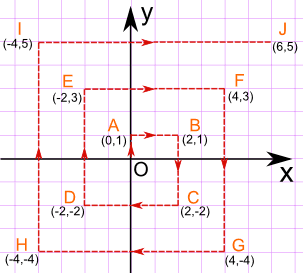Now it is just a matter of finding the distance between two points

The distance between the points (xA,yA) and (xB,yB) is given by the formula: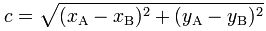and one of those points is always the origin, which is at (0,0), so when xB and yB are zero we get: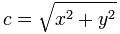Example, for the point E (-2, 3), x = -2 and y = 3, and so:Hopefully that will help make your job easier.## Direction?

There is one more thing Jade must know: to get back to the plane, what compass bearing must he travel?

This will be covered in Activity: A Walk in the Desert 2